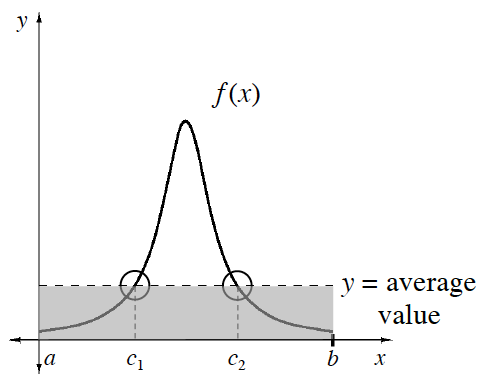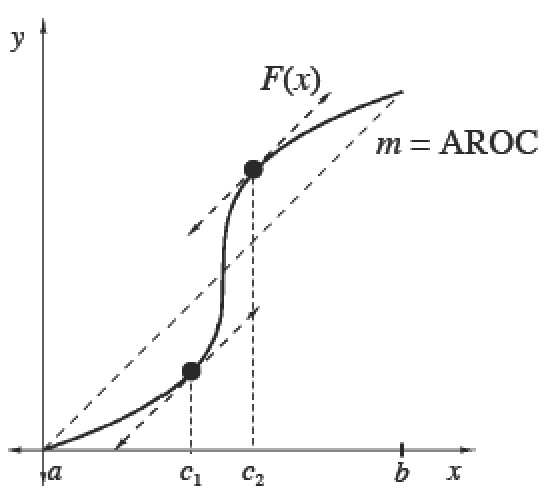### Home > APCALC > Chapter 7 > Lesson 7.1.2 > Problem7-18

7-18.

It just so happens that Hustling Harry’s grade in math class at any week $t$ during the semester is calculated by $g(t)=46\cos(\frac{t}{10})+10\sin(\frac{t}{2})+40$. At what point during the $18$-week semester is Harry’s grade equal to his average grade for the semester?

This is an application of the Mean Value Theorem.

Show setup and steps. $t ≈7.630$ weeks

The Mean Value Theorem

 The Mean Value Theorem for IntegralsIf $f$ is continuous on $[a, b]$, then there exists at least one point $x = c$ in $\left(a, b\right)$ such that $\frac { 1 } { b - a } \int _ { a } ^ { b } f ( x ) d x= f(c)$.The Mean Value Theorem for DerivativesIf $F$ is continuous on $[a, b]$ and differentiable on $\left(a, b\right)$, then there exists at least one point $x = c$ in $\left(a, b\right)$ such that $F ^ { \prime } ( c ) = \frac { F ( b ) - F ( a ) } { b - a } = f ( c )$.